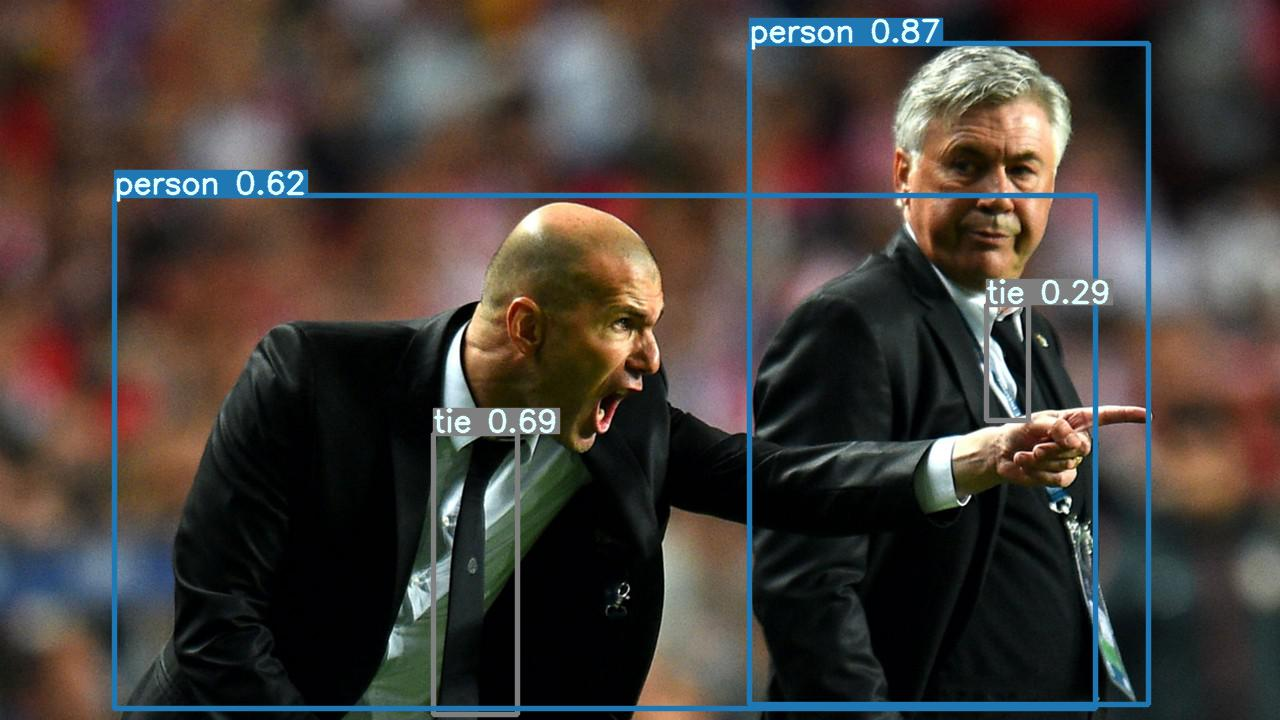# Confidential AI - Algorithms

## Introduction

This article describes the various algorithms supported by Confidential AI. It also lists the modes and data types supported by each algorithm.

## Algorithms

Algorithm Modes Problem Supported Datatype Supported
Decision Trees Inference and Learning

Classification

Regression
Tabular
KNN (K-Nearest Neighbor) Inference and Learning

Classification

Tabular
Logistic Regression Inference and Learning

Regression

Tabular
SVM (Support Vector Machines) Inference and Learning

Classification

Regression

Tabular
Yolov5 Inference only Object Detection Images
ResNet50 Inference only Object Recognition Images

Classification

Regression
Tabular
Gradient-Boosted Random Forest Inference and Learning

Classification

Regression
Tabular

### Decision Trees

The decision tree implementations provide a decision tree classifier and regression classes, capable of performing multi-class classification and applied to regression problems. The method uses a tree-like model of decisions and their possible consequences to predict the value of a target variable.

### K-Nearest Neighbor

The k-nearest neighbor implementation provides a supervised neighbors-based learning method for classification. The classifier implements learning based on the k-nearest neighbors of each query point, where k is an integer value specified by the user.

### Logistic Regression

Logistic regression is a statistical method for predicting certain classes or events existing such as pass/fail, high/medium/low, legit/fraud, healthy/unhealthy using a logistic function. The model is used in various fields, including the financial and healthcare sectors to predict the risk involved in the transaction, or to predict the risk of developing a given disease respectively.

#### References

https://en.wikipedia.org/wiki/Logistic_regression

https://scikit-learn.org/stable/modules/linear_model.html#logistic-regression

### Support Vector Machines (SVM)

Support Vector Machines are supervised learning methods that analyze data used for classification, or regression in tasks such as outliers detection. Support Vector Classification (SVC) can efficiently perform both linear and non-linear classification, as it supports high dimensional spaces. The method of SVC can be extended to solve regression problems with the implemented method of Support Vector Regression (SVR).

#### References

https://en.wikipedia.org/wiki/Support-vector_machine

https://scikit-learn.org/stable/modules/svm.html

### YOLO version 5

YOLO which means 'You only look once' is an object detection algorithm that divides images into a grid system. Each cell in the grid is responsible for detecting objects within itself. YOLOv5 is the latest release of the YOLO family of object detection algorithms trained on the COCO dataset and open-source research activity by Ultralytics.

Use the YOLOv5 implementation of Fortanix Confidential AI to securely detect objects in the input images, without revealing the input dataset or the objects detected. Detected objects will be shown inside boxes and the inferred label and accuracy of detection will be displayed.#### References

https://docs.ultralytics.com/

### Deep Residual Networks (ResNet50)

ResNet50 is a convolutional neural network that is 50 layers deep, trained by NVIDIA.

With more layers to the residual learning neural network, shortcut identity mappings are used to

improve the error rate of the model.

The ResNet50 implementation of Fortanix Confidential AI is able to securely identify objects, without revealing the input dataset or the objects identified.

The detected objects will be provided in a tabular output file that contains the image name, the object identified, and the accuracy of identification.

#### References

https://iq.opengenus.org/resnet50-architecture/

https://viso.ai/deep-learning/resnet-residual-neural-network/

### XGBoost - Gradient-Boosted Decision Trees/Random Forests

Standard Decision Trees use a True-False pattern for classification or regression in a chain of questions, whereas Gradient-Boosted Decision Trees use an ensemble learning algorithm. This system uses multiple decision trees with subsets of data, essentially using multiple weak tree models to create a stronger final model.

The Random Forest approach uses random bagging in order to create the initial tree subsets, but overall is similar, differing in the way the final results are put together.

Fortanix utilizes the XGBoost family of algorithms.

#### References

https://www.nvidia.com/en-us/glossary/data-science/xgboost/